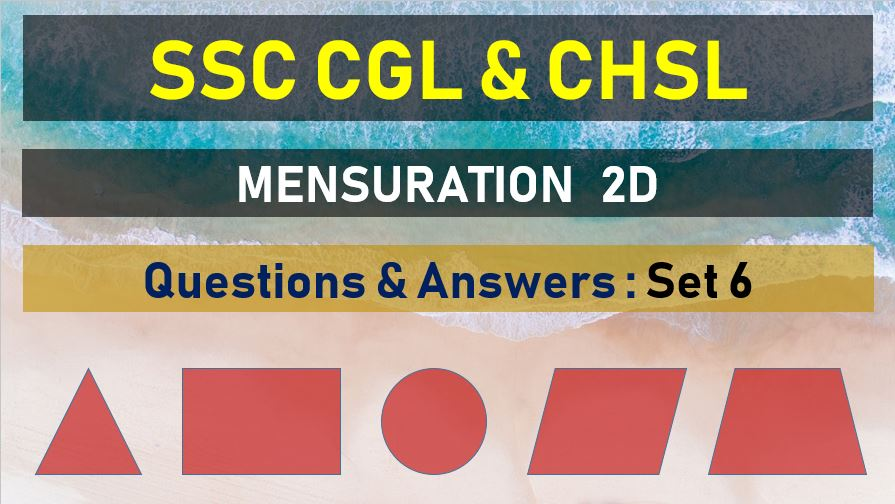Friday, May 7, 2021
Home > mensuration > SSC CHSL Area and Perimeter Questions Answers Set 6

# SSC CHSL Area and Perimeter Questions Answers Set 6SSC Mensurations 2D
Area and Perimeter Questions Sets
Set 1Set 2Set 3Set 4Set 5
Set 6Set 7Set 8Set 9Set 10

SSC CHSL Area and Perimeter Questions Answers Set 6: Ques No 1

The diameter of a toy wheel is 14cm, What is the distance travelled by it in 15 revolutions?

Options:

A. 880 cm

B. 660 cm

C. 600 cm

D. 560 cm

Solution:

SSC CHSL Area and Perimeter Questions Answers Set 6: Ques No 2

A can go round a circular path 8 times in 40 minutes. If the diameter of the circle is increased to 10 times the original diameter, the time required by A to go round the new path once travelling at the same speed as before is

Options:

A. 25 min

B. 20 min

C. 50 min

D. 100 min

Solution:

SSC CHSL Area and Perimeter Questions Answers Set 6: Ques No 3

The base of a triangle is 15 cm and its height is 12 cm and the height of another triangle of double the area having the base 20 cm is

Options:

A. 9 cm

B. 18 cm

C. 8 cm

D. 12.5 cm

Solution:

SSC CHSL Area and Perimeter Questions Answers Set 6: Ques No 4

If a wire is bent into the shape of a square, the area of the square is 81sq. cm, When the wire is bent into a semicircular shape, the area of the semicircle is : (use pie= 22/7)

Options:

A. 154 sq. cm

B. 77 sq. cm

C. 44 sq. cm

D. 22 sq. cm

Solution:

SSC CHSL Area and Perimeter Questions Answers Set 6: Ques No 5

If the area of a triangle with base 12 cm is equal to the area of square with side 12 cm, the altitude of the triangle will be

Options:

A. 12 cm

B. 24 cm

C. 18 cm

D. 36 cm

Solution:

SSC CHSL Area and Perimeter Question Answers Set 6: Ques No 6

The sides of a triangle are 3cm, 4 cm and 5 cm. The area (in cm2) of the triangle formed by joining the mid points of this triangle is

Options:

A. 6

B. 3

C. 3/2

D. 3/4

Solution:

SSC CHSL Area and Perimeter Question Answers Set 6: Ques No 7

Three circle of radius 3.5 cm each are placed in such a way that each touches the other two. The area of portion enclosed by the circles is

Options:

A. 1.975 cm2

B. 1.967 cm2

C. 19.68 cm2

D. 21.22 cm2

Solution:

SSC CHSL Area and Perimeter Question Answers Set 6: Ques No 8

The area of a circular garden is 2464 sq. m. How much distance will have to be covered if you like to cross the garden along its diameter? (use pie = 22)

Options:

A. 56 m

B. 48 m

C. 28 m

D. 24 m

Solution:

SSC CHSL Area and Perimeter Question Answers Set 6: Ques No 9

Four equal circles each of radius ‘a’ units touch one another. The area enclosed between them (pie= 22/7). In square units, is

Options:

A. 3a2

B. 6a2/7

C. 41a2

D. a2/7

Solution:

SSC CHSL Area and Perimeter Question Answers Set 6: Ques No 10

The area of the greatest circle inscribed inside a square of side 21 cm is (take pie =22/7)

Options:

A. 703/2 cm2

B. 701/2 cm2

C. 693/2 cm2

D. 695/2 cm2